• Range: Max - min
• Variance: roughly the average squared deviation from the mean
• It is calculated using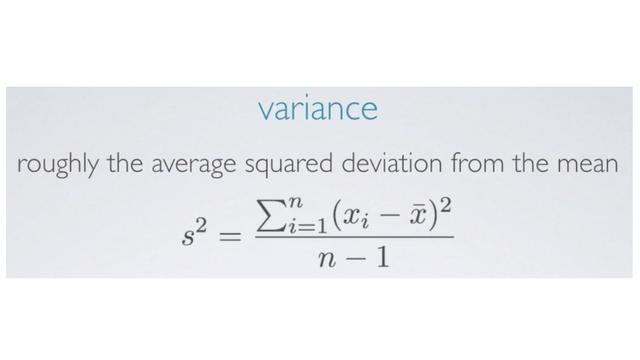• Sample variance is denoted using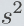• Population variance is denoted using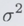• Standard Deviation: roughly the average deviation around the mean, and has the same units as the ata
• It is square root of the variance:• Sample sd is denoted using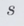• Population sd is denoted using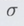• Inter-quartile range (IQR): range of the middle 50% of the data, distance between the first quartile (25th percentile) and third quartile (75th percentile)
• IQR = Q3 - Q1
• Example: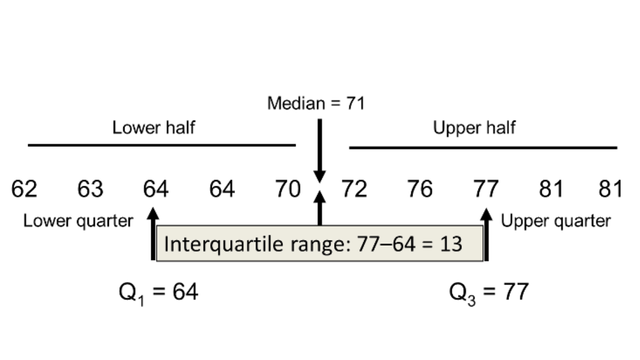The value of the IQR itself, the number 12, isn't as informative on its own, but would be very useful when comparing two distributions. The reason why the IQR is a more reliable measure of spread in sample data than the range, which we said was the difference between the maximum and the minimum. Is that it doesn't rely on the end points, which may be unusual observations or potential outliers. Instead, it measures the variability for the bulk of the data around the center.

• N/A